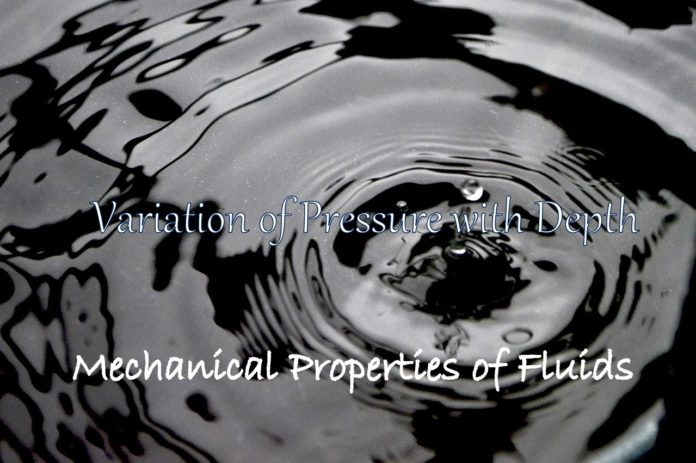Consider a fluid at rest in a container. In Fig.1 point 1 is at height h above a point 2.The pressures at points 1 and 2 are P1 and P2 respectively. Consider a cylindrical element of fluid having area of base A and height h. As the fluid is at rest the resultant horizontal forces should be zero and the resultant vertical forces should balance the weight of the element. The forces acting in the vertical direction are due to the fluid pressure at the top (P1A) acting downward, at the bottom (P2A) acting upward. If mg is weight of the fluid in the cylinder we have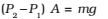Now, if ρ is the mass density of the fluid, we have the mass of fluid to be m = ρ V = ρ h A so that,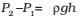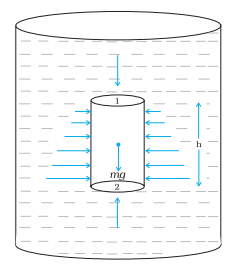Fig.1 Fluid under gravity. The effect of gravity is illustrated through pressure o a vertical cylindrical column.

Pressure difference depends on the vertical distance h between the points (1 and 2), mass density of the fluid ρ and acceleration due to gravity g. If the point 1 under discussion is shifted to the top of the fluid (say water), which is open to the atmosphere, P1 may be replaced by atmospheric pressure (Pa) and we replace P2 by P.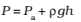Thus, the pressure P, at the depth below the surface of a liquid open to the atmosphere is greater than atmospheric pressure by an amount ρgh. The excess of pressure, P –Pa, at depth h is called a gauge pressure at that point.

The area of the cylinder is not appearing in the expression of absolute pressure in Eq. (above). Thus, the height of the fluid column is important and not cross sectional or base area or the shape of the container. The liquid pressure is the same at all points at the same horizontal level (same depth). The result is appreciated through the example of a hydrostatic paradox.